# Front

### You have 3 tries to reach the target.

###### Important: play with 3 identical golf balls

Take 3 balls and place them as indicated below. Play and keep score. Place a circular object in the center of the hole, the size of the orange circle so you can have a physical entity to hit with the ball.

#### 4 PointsPlace the ball 3 feet from the hole on the higher black dot  (S). Use the dotted line for help to putt so that the ball rolls over the hole and comes to rest in the Welling-putt zone (F).

#### 3 Points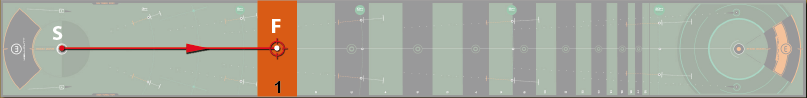1. Place the ball on the hole line.
2. Putt towards zone 1. (F)

#### 4 Points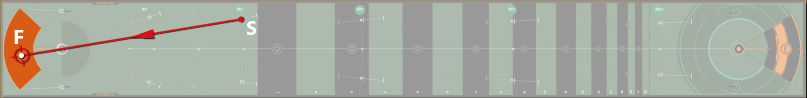Place the ball 6 feet from the hole on the higher black dot (S). Use the dotted line for help to putt so that the ball rolls over the hole and comes to rest in the Welling-putt zone (F).

#### 3 Points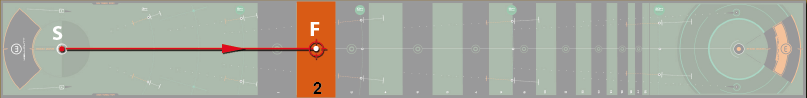1. Place the ball on the hole line.
2. Putt towards zone 2. (F).

#### 4 PointsPlace the ball 10 feet from the hole on the higher black dot  (S). Use the dotted line for help to putt so that the ball rolls over the hole and comes to rest in the Welling-putt zone (F)

#### 4 Points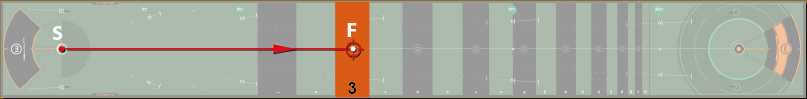1. Place the ball on the hole line.
2.  Putt towards zone 3. (F).

#### 5 Points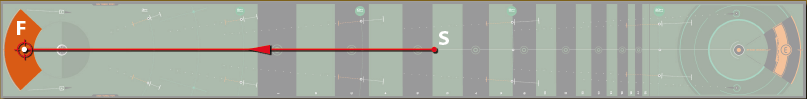Place the ball on the white line at the 12ft intersection in the black area (S).  You have to go over the hole and end up in the welling-putt zone (F).

#### 3 Points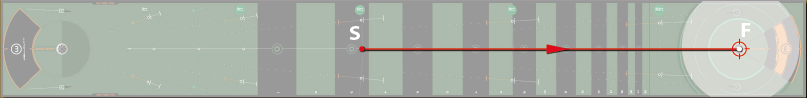Place the ball in the green line in zone 3 (S).  Putt and end up in the largest circle around the hole (F).

#### 5 Points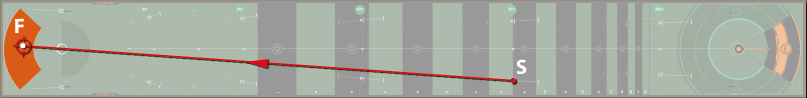Place the ball 15 feet from the hole on the lower black dot (S). Use the dotted line for help to putt so that the ball rolls over the hole and comes to rest in the Welling-putt zone (F)

##### “When you get to 22 points, you can move on to the back nine.”# Back

### You have 3 tries to reach the target.

#### 5 Points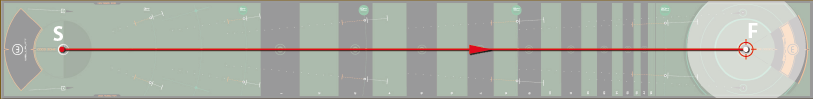Place the ball on the hole line. (S). Putt and end up in the largest circle around the hole (F).

#### 3 Points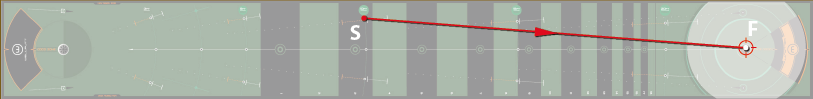Place the ball 10 feet from the hole on the black dot (S). Putt and end up in the largest circle around the hole (F).

#### 5 Points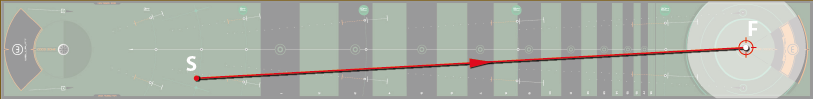Place the ball on the green line 4.5feet from the hole. (S). Putt and end up in the largest circle around the hole (F).

#### 3 PointsPlace the ball 10 feet from the hole on the white line (S) Putt and end up in the middle circle around the hole (F).

#### 5 Points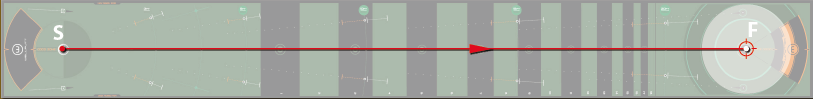Place the ball on the hole line. (S). Putt and end up in the middle circle around the hole (F).

#### 4 PointsPlace the ball at 4.5 feet on the central dot. (S).  Putt and end up in the middle circle around the hole (F).

#### 5 Points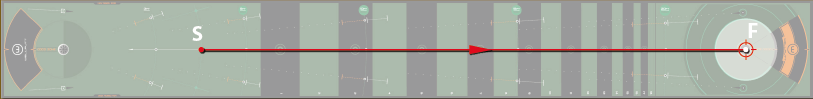Place the ball at 4.5 feet on the central dot. (S).  Putt and end up in the smallest circle around the hole (F).

#### 3 PointsPlace the ball 10 feet from the hole on the white line ( S). Putt and end up in the smallest circle around the hole (F).

#### 5 Points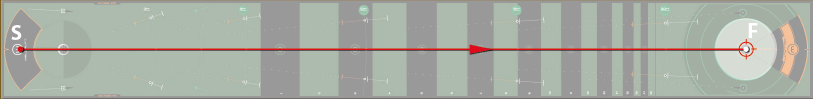Place the ball on the welling-putt zone. (S).  Putt and end up in the smallest circle around the hole (F).

##### “When you reach 47 points, you can move on to the blue course.”